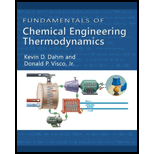Chapter 3.9, Problem 26P### Fundamentals of Chemical Engineeri...

1st Edition
Kevin D. Dahm + 1 other
ISBN: 9781111580704

#### Solutions

Chapter
Section### Fundamentals of Chemical Engineeri...

1st Edition
Kevin D. Dahm + 1 other
ISBN: 9781111580704
Textbook Problem

# 1 kg of nitrogen is contained in a piston-cylinder device. The nitrogen is isothermally compressed from P = 1 bar to P = 10 bar at T = 300 K. Find the initial volume, final volume, and work and heat added to or removed from the nitrogen. A. Assume nitrogen behaves as an ideal gas. B. Use Figure 2-3 as much as possible. If you need an equation of state, use the van der Waals equation, with a = 1.37 × 106 bar · cm6/mol2 and b = 38.6 cm3/mol. Problems 3-27 through 3-29 involve the same compound that was considered in Chapter 2, Problems 2-22 through 2-26: A molecular weight of 50 lb/lb-mol A normal melting point of 50°F A normal boiling point of 150°F A constant heat capacity in the solid phase of CP = 0.7 BTU/lb·°R A constant heat capacity in the liquid phase of CP = 0.85 BTU/lb· °R A constant density in the solid phase of ρ = 40 lb/ft3 A constant density in the liquid phase of ρ = 30 lb/ft3 An ideal gas heat capacity of C P * = 1 − T/1200 + T2 × 10−6 BTU/lb·°R, where T is the temperature in °R An enthalpy of fusion Δ H ^ fus = 75 BTU/lb at atmospheric pressure An enthalpy of vaporization Δ H ^ vap = 125 BTU/lb at atmospheric pressure

(A)

Interpretation Introduction

Interpretation:

Determine the initial volume, final volume, work and heat transfer.

Concept Introduction:

Write the expression to calculate the number of moles in nitrogen.

N=MMW

Here, molecular weight of nitrogen is MW and mass of nitrogen is M.

Write the expression to calculate the initial volume.

Vinitial=NRTinitialPinitial

Here, initial temperature and pressure is Tinitial and Pinitial, number of moles is N, and gas constant is R.

Write the expression to calculate the final volume.

Vfinal=NRTfinalPfinal

Here, final temperature and pressure is Tfinal and Pfinal respectively.

Write the energy balance around the compressor.

ddt{M(U^+v22+gh)}=m˙in(H^in+vin22+ghin)m˙out(H^out+vout22+ghout)+WS+WEC+Q

Here, time is t, total mass of the system is M, specific internal energy of the system is U^, velocity of the system is v, height of the system is h, acceleration due to gravity is g, individual quantities of mass added to and removed from the process is m˙in and m˙out, specific enthalpies of streams inlet and outlet is H^in and H^out, heights at which streams enters and leave the system is hin and hout, total expansion or contraction work added throughout the process is WEC, total shaft work added throughout the process is WS, and the total heat added throughout the process is Q.

Explanation

Calculate the number of moles in nitrogen.

N=MMW        (1)

Substitute 1 kg for M and 28.02g/mol for MW in Equation (1).

N=1kg28.02g/mol

Calculate the initial volume.

Vinitial=NRTinitialPinitial        (2)

Substitute 1 bar for Pinitial, 250 K for Tinitial, 83.14barcm3molK for R, and 1kg28.02g/mol for N in Equation (2).

Vinitial=(1kg28.02g/mol)(83.14barcm3molK)250K1bar=(1kg28.02g/mol)(1,000g1kg)(83.14barcm3molK)250K1bar=741,800cm3

Thus, the initial volume is 741,800cm3.

Calculate the final volume.

Vfinal=NRTfinalPfinal        (3)

Substitute 10 bar for Pfinal, 250 K for Tfinal, 83.14barcm3molK for R, and 1kg28.02g/mol for N in Equation (3).

Vfinal=(1kg28.02g/mol)(83.14barcm3molK)250K10bar=(1kg28.02g/mol)(1,000g1kg)(83.14barcm3molK)250K1bar=74,180cm3

Thus, the initial volume is 74,180cm3.

Calculate the energy balance around the compressor

(B)

Interpretation Introduction

Interpretation:

Determine the initial volume, final volume, work and heat transfer.

Concept Introduction:

Write the expression to calculate the initial molar volume by using the van der Waals equation.

Pinitial=RTinitialV_initialbaV_initial2

Here, constants are a and b respectively.

Write the expression to calculate the initial volume.

V=MV_MW

Write the expression to calculate the final molar volume by using the van der Waals equation.

Pfinal=RTfinalV_finalbaV_final2

Write the expression to calculate the final volume.

V=MV_finalMW

### Still sussing out bartleby?

Check out a sample textbook solution.

See a sample solution

#### The Solution to Your Study Problems

Bartleby provides explanations to thousands of textbook problems written by our experts, many with advanced degrees!

Get Started

## Additional Engineering Solutions

#### What is physical independence?

Database Systems: Design, Implementation, & Management

#### What is residual risk?

Principles of Information Security (MindTap Course List)

#### Explain the use of permissions on a network.

Enhanced Discovering Computers 2017 (Shelly Cashman Series) (MindTap Course List)